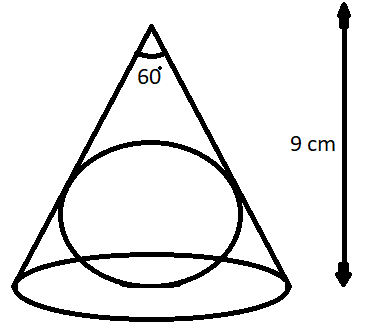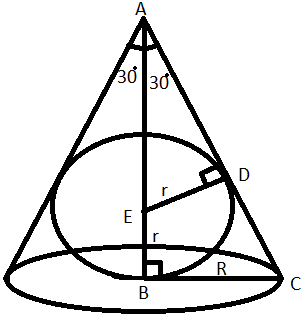QuestionAnswers

# A sphere is placed in a cone of height 9cm as shown in the figure. The volume of the sphere, if it touches lateral surface and base of the cone is$\left( a \right)3\pi {\text{ c}}{{\text{m}}^3}$$\left( b \right)12\pi {\text{ c}}{{\text{m}}^3}$$\left( c \right)24\pi {\text{ c}}{{\text{m}}^3}$$\left( d \right)36\pi {\text{ c}}{{\text{m}}^3}$Verified
128.4k+ views
Hint: In this particular question use the concept that if two triangles are similar to each other than the ratio of their respective sides is always equal and use the concept in a right angle triangle tan x is the ratio of perpendicular side to base side length so use these concepts to reach the solution of the question.Given data:
Height of the cone is 9cm.
Therefore, AB = 9cm.
From the figure it is given that the central angle is 60 degrees.
So AB is the bisector of central angle, therefore, $\angle BAC = \dfrac{{{{60}^o}}}{2} = {30^o}$
Now as we know that the radius of the sphere with the tangent always makes a 90 degrees.
So in the above figure AC is the tangent for the internal sphere. So it makes a 90 degrees with the radius of the sphere as shown in the above figure.
Let the radius of the sphere be r cm and the radius of the cone be R cm, as shown in the above figure.
Now in triangle ABC and ADE
$\angle ABC = \angle ADE = {90^o}$
$\angle BAC = \angle EAD = {30^o}$
$\angle ACB = \angle AED = {180^o} - {90^o} - {30^o} = {60^o}$
So by AAA congruency triangle ABC and triangle ADE are similar to each other.
Now as we know that if two triangles are similar to each other then the ratio of their respective sides is always equal.
$\Rightarrow \dfrac{{AE}}{{AC}} = \dfrac{{AD}}{{AB}} = \dfrac{{DE}}{{BC}}$
Now substitute the values we have,
$\Rightarrow \dfrac{{AE}}{{AC}} = \dfrac{{AD}}{9} = \dfrac{r}{R}$
$\Rightarrow \dfrac{{AE}}{{AC}} = \dfrac{r}{R}$.............. (1)
Now from the figure, AB = AE + EB, but EB is also the radius of the sphere.
Therefore, AB = AE + r
Therefore, AE = AB – r = 9 – r.............. (2)
Now in triangle ABC we have,
$\tan {30^o} = \dfrac{{{\text{perpendicular}}}}{{{\text{base}}}} = \dfrac{R}{{AB}}$
$\Rightarrow R = \dfrac{9}{{\sqrt 3 }}$.......................... (3), $\left[ {\because \tan {{30}^o} = \dfrac{1}{{\sqrt 3 }}} \right]$
Now apply Pythagoras theorem in triangle ABC we have,
$\Rightarrow {\left( {{\text{Hypotenuse}}} \right)^2} = {\left( {{\text{perpendicular}}} \right)^2} + {\left( {{\text{base}}} \right)^2}$
$\Rightarrow {\left( {{\text{AC}}} \right)^2} = {\left( {{\text{AB}}} \right)^2} + {\left( {{\text{BC}}} \right)^2}$
$\Rightarrow {\left( {{\text{AC}}} \right)^2} = {\left( {\text{9}} \right)^2} + {\left( {\text{R}} \right)^2}$
$\Rightarrow {\text{AC}} = \sqrt {{{\left( {\text{9}} \right)}^2} + {{\left( {\text{R}} \right)}^2}}$..................... (4)
Now substitute the value from equation (2), (3) and (4) in equation (1) we have,
$\Rightarrow \dfrac{{9 - r}}{{\sqrt {{{\left( 9 \right)}^2} + {R^2}} }} = \dfrac{r}{R}$
$\Rightarrow \dfrac{{9 - r}}{{\sqrt {{{\left( 9 \right)}^2} + {{\left( {\dfrac{9}{{\sqrt 3 }}} \right)}^2}} }} = \dfrac{r}{{\dfrac{9}{{\sqrt 3 }}}}$
Now simplify we have,
$\Rightarrow \dfrac{{9 - r}}{{9\sqrt {1 + \dfrac{1}{3}} }} = \dfrac{r}{{\dfrac{9}{{\sqrt 3 }}}}$
$\Rightarrow \dfrac{{9 - r}}{{9\sqrt {\dfrac{4}{3}} }} = \dfrac{r}{{\dfrac{9}{{\sqrt 3 }}}}$
$\Rightarrow \dfrac{{9 - r}}{{2\left( {\dfrac{9}{{\sqrt 3 }}} \right)}} = \dfrac{r}{{\dfrac{9}{{\sqrt 3 }}}}$
$\Rightarrow 9 - r = 2r$
$\Rightarrow 3r = 9$
$\Rightarrow r = \dfrac{9}{3} = 3$cm.
Now as we know the volume of the sphere is given as, $\dfrac{4}{3}\pi {r^3}$ cubic units, where r is the radius of the sphere.
Therefore, volume of sphere is = $\dfrac{4}{3}\pi {\left( 3 \right)^3} = 36\pi {\text{ c}}{{\text{m}}^3}$
So this is the required volume of the sphere.

So, the correct answer is “Option D”.

Note: Whenever we face such types of questions the key concept we have to remember is that always recall the Pythagoras theorem and the formula of the volume of the sphere which is all stated above, so first find out the radius of the sphere as above, then use the formula of volume of the sphere and simplify as above, we will get the required volume of the sphere.